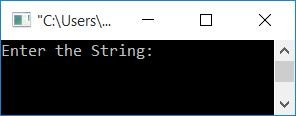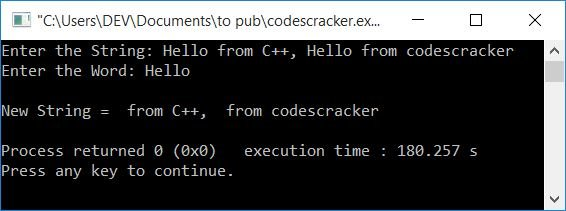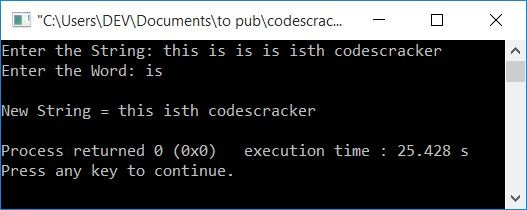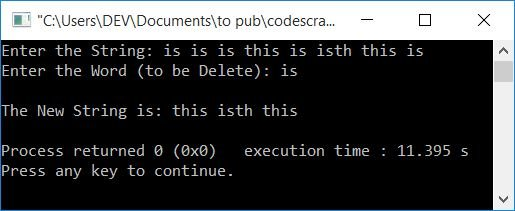# C++ Program to Delete a Word from a String

In this article, you will learn and get code to delete (or remove) a word from a string in C++. Both words and strings must be entered by the user at runtime.

Here is the list of programs you will go through:

The first program has the limitation that if the user enters the string this is codescracker and the word to delete as is. Then is from this also gets deleted. So the new string will be th codescracker. To overcome this problem, we've modified this program.

The second program (the modified program) also removes extra spaces from the new string.

## Delete a word from a string

To delete any desired word from a given string in C++ programming, you have to ask the user to enter the string and word. Then delete the entered word (along with its duplicate) from the entered string.

The question is, "Write a program in C++ that deletes a word from a string." Here is its answer:

```#include<iostream>
#include<stdio.h>
#include<string.h>
using namespace std;
int main()
{
char str, wrd;
int i, j, strLen, wrdLen, tmp, chk=0;
cout<<"Enter the String: ";
gets(str);
cout<<"Enter the Word: ";
cin>>wrd;
strLen = strlen(str);
wrdLen = strlen(wrd);
for(i=0; i<strLen; i++)
{
tmp = i;
for(j=0; j<wrdLen; j++)
{
if(str[i]==wrd[j])
i++;
}
chk = i-tmp;
if(chk==wrdLen)
{
i = tmp;
for(j=i; j<(strLen-wrdLen); j++)
str[j] = str[j+wrdLen];
strLen = strLen-wrdLen;
str[j]='\0';
}
}
cout<<"\nNew String = "<<str;
cout<<endl;
return 0;
}```

This program was built and runs under the Code::Blocks IDE. Here is its sample run:Now supply any string, say Hello from C++, Hello from codescracker and a word say Hello. Press the `ENTER` key to delete Hello from the entered string as shown in the snapshot given below:The following is the dry run of the above program with user input as provided in the above sample run:

• Initial value, chk=0
• When the user enters the string Hello from C++, Hello from codescracker, then it gets stored in str in a way that:
• str=H
• str=e
• str=l
• and so on.
• Similarly, the entered word "Hello" gets stored in wrd in a way that:
• wrd=H
• wrd=e
• and so on.
• The function strlen() takes a string as its argument and returns the length of the string.
• So strLen holds the length of the string, whereas wrdLen holds the length of the word. That is, strLen=39 and wrdLen=5.
• Now program flow starts evaluating the for loop.
• For the first time, the initialization part gets executed, and it executes only once.
• So i=0 and the condition i<strLen or 0<39 evaluates to be true; therefore program flow goes inside the loop. The value of i is incremented and the condition is evaluated the next time. Every time program flow goes inside the loop until the condition evaluates to be false.
• Now inside the loop, the value of i (0) gets initialized to tmp.
• There is another for loop from here.
• So 0 gets initialized to j and the condition j<wrdLen or 0<5 evaluates to be true, therefore, program flow goes inside the loop and the condition str[i]==wrd[j] or str==wrd or H==H evaluates to be true, therefore the value of i gets incremented. So i=1
• Now the value of j gets incremented. So j=1. Then the condition, j<wrdLen or 1<5, evaluates to be true, therefore program flow again goes inside the loop and the condition, str[i]==wrd[j] or str==wrd or e==e, evaluates to be true, therefore the value of i again gets incremented.
• This procedure is repeated until the condition of this for loop evaluates to false.
• Because the word gets matched with the first word of the string, that is, Hello, with character-by-character matching.
• So the value of i will be 5 after exiting from this loop, and i-tmp or 5-0 or 5 gets initialized to chk. Here 0 (tmp's value) is the previous value of i. That is, the value before the inner for loop begins.
• This statements checks whether each and every character of the word gets matched with each and every character of the string (starts from the index, before this loop).
• Now, because the value of chk is equal to the value of wrdLen, simply shift all the characters that follows the matched word (in the string) back to the 5 index. Because 5 is the length of the word.
• As a result, the new string will be from C++, Hello from codescracker after executing this if's block of code.
• Don't forgot to initialize a null-terminated character after the last character's index.
• Now program flow again goes back to the outer for loop, increments the value of i, and evaluates the condition.
• This process continues until the condition is evaluated as false.
• After exiting from the loop, just print the value of str.

Note: If you want to create the same program without using strlen(), then you can refer to Count Occurrence of a Word in a String. I know the program is not the same, but that article is a little similar to this one. Without using any string functions, we counted the desired word from the string.So you can refer to that article as an idea that you can implement yourself. That will be more helpful for you.

### Modified Version of the Previous Program

Here is the complete version of the previous program that deletes a word from a string. This program also removes extra spaces from the new string.

```#include<iostream>
#include<stdio.h>
#include<string.h>
using namespace std;
int main()
{
char str, wrd;
int i, j, strLen, wrdLen, tmp, chk=0;
int doIncrement, isSpace;
cout<<"Enter the String: ";
gets(str);
cout<<"Enter the Word: ";
cin>>wrd;
strLen = strlen(str);
wrdLen = strlen(wrd);
for(i=0; i<strLen; i++)
{
tmp = i;
doIncrement = 0;
for(j=0; j<wrdLen; j++)
{
if(str[i]==wrd[j])
{
if(tmp>0 && (tmp+wrdLen)<strLen)
{
if(str[tmp-1]== ' ' && str[tmp+wrdLen]==' ')
doIncrement=1;
}
else if(tmp==0 && (tmp+wrdLen)<strLen)
{
if(str[tmp+wrdLen]==' ')
doIncrement=1;
}
else if(tmp>0 && (tmp+wrdLen)==strLen)
{
if(str[tmp-1]== ' ')
doIncrement=1;
}
if(doIncrement==1)
i++;
else
break;
}
}
chk = i-tmp;
if(chk==wrdLen)
{
i = tmp;
for(j=i; j<(strLen-wrdLen); j++)
str[j] = str[j+wrdLen];
strLen = strLen-wrdLen;
i = tmp;
str[j]='\0';
}
}
strLen = strlen(str);
i=0;
while(str[i]!='\0')
{
isSpace = 0;
if(str[i]==' ' && str[i+1]==' ')
{
for(j=i; j<(strLen-1); j++)
{
str[j] = str[j+1];
isSpace = 1;
}
}
if(i==0 && str[i]==' ')
{
for(j=i; j<(strLen-1); j++)
{
str[j] = str[j+1];
isSpace = 1;
}
}
if(isSpace==0)
i++;
else
{
str[j]='\0';
strLen--;
}
}
cout<<"\nNew String = "<<str;
cout<<endl;
return 0;
}```

Here's an example run with user input; this is is is isth codescracker as a string, and is as the word to delete:### In C++, delete a word from a string using a two-dimensional array

Using a two-dimensional array, the program becomes easier to create or understand. Here is the program:

```#include<iostream>
#include<stdio.h>
#include<string.h>
using namespace std;
int main()
{
char str, wrd, strInTwoDArray;
int i, j=0, k=0;
cout<<"Enter the String: ";
gets(str);
cout<<"Enter the Word (to be Delete): ";
gets(wrd);
for(i=0; str[i]!='\0'; i++)
{
if(str[i]==' ')
{
strInTwoDArray[k][j]='\0';
k++;
j=0;
}
else
{
strInTwoDArray[k][j]=str[i];
j++;
}
}
strInTwoDArray[k][j] = '\0';
j=0;
for(i=0; i<(k+1); i++)
{
if(!strcmp(strInTwoDArray[i], wrd))
strInTwoDArray[i][j]='\0';
}
cout<<"\nThe New String is: ";
j=0;
for(i=0; i<(k+1); i++)
{
if(strInTwoDArray[i][j] == '\0')
continue;
else
cout<<strInTwoDArray[i];
}
cout<<endl;
return 0;
}```

Here's a sample run with user input: is is is this is isth this is (as a string) and is (as a word):#### The same program in different languages

C++ Quiz

« Previous Program Next Program »